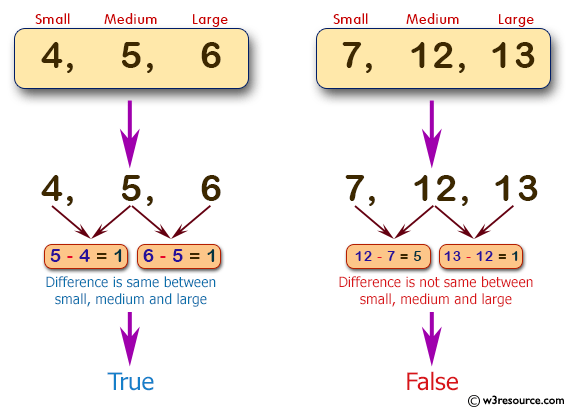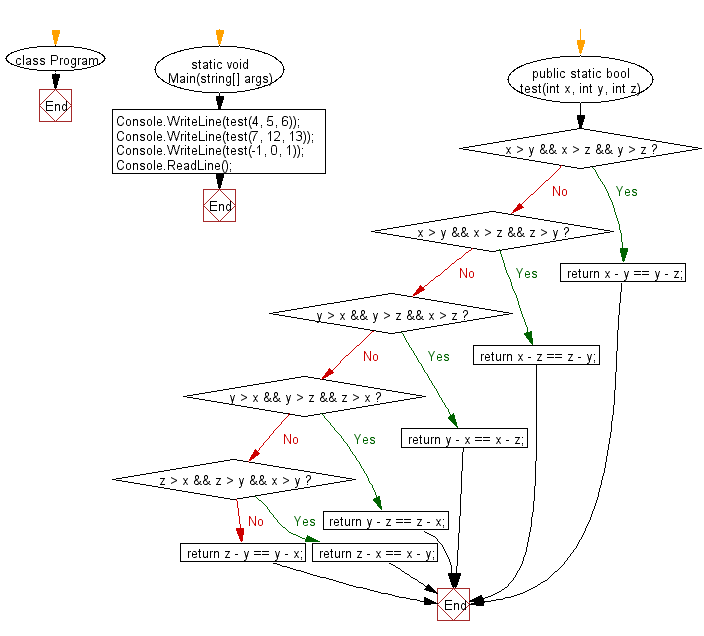﻿ C# - Difference between small, medium, medium of 3 integers# C# Sharp Basic Algorithm Exercises: Check three given integers and return true if the difference between small and medium and the difference between medium and large is same

## C# Sharp Basic Algorithm: Exercise-59 with Solution

Write a C# Sharp program to check three given integers (small, medium and large) and return true if the difference between small and medium and the difference between medium and large is same.

Pictorial Presentation:Sample Solution:-

C# Sharp Code:

``````using System;
using System.Collections.Generic;
using System.Linq;
using System.Text;
namespace exercises
{
class Program
{
static void Main(string[] args)
{
Console.WriteLine(test(4, 5, 6));
Console.WriteLine(test(7, 12, 13));
Console.WriteLine(test(-1, 0, 1));
}
public static bool test(int x, int y, int z)
{
if (x > y && x > z && y > z) return x - y == y - z;
if (x > y && x > z && z > y) return x - z == z - y;
if (y > x && y > z && x > z) return y - x == x - z;
if (y > x && y > z && z > x) return y - z == z - x;
if (z > x && z > y && x > y) return z - x == x - y;
return z - y == y - x;
}
}
}
```
```

Sample Output:

```True
False
True```

Flowchart:C# Sharp Code Editor:

Improve this sample solution and post your code through Disqus

What is the difficulty level of this exercise?

Test your Programming skills with w3resource's quiz.

﻿